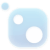# Top 20 NuGet gmp Packages## The C++ Mathematical Expression Toolkit Library (ExprTk)

The C++ Mathematical Expression Toolkit Library (ExprTk) is a simple to use, easy to integrate and extremely efficient run-time mathematical expression parsing and evaluation engine. The parsing engine supports numerous forms of functional and logic processing semantics and is easily extendible.
The GMP Native Interface for .NET Library exposes to .NET (through P-Invoke and .NET types) all of the functionality of the GNU MP Library (version 6.1.2). It automatically loads at runtime the 32-bit or 64-bit GNU MP library that matches the current CPU architecture, thus allowing building Visual S...
Mpir.NET lets you use the MPIR library, which is a GMP fork for Windows, from .NET languages. The current version incorporates 32- and 64-bit builds of MPIR 2.7.0.
The C++ Mathematical Expression Toolkit Library (ExprTk) Extra Examples
GMP is a free library for arbitrary precision arithmetic, operating on signed integers, rational numbers, and floating-point numbers.
MPIR is a library for arbitrary precision arithmetic, operating on signed integers, rational numbers, and floating point numbers. It has a rich set of functions, and the functions have a regular interface
MPIR is a library for arbitrary precision arithmetic, operating on signed integers, rational numbers, and floating point numbers. It has a rich set of functions, and the functions have a regular interface